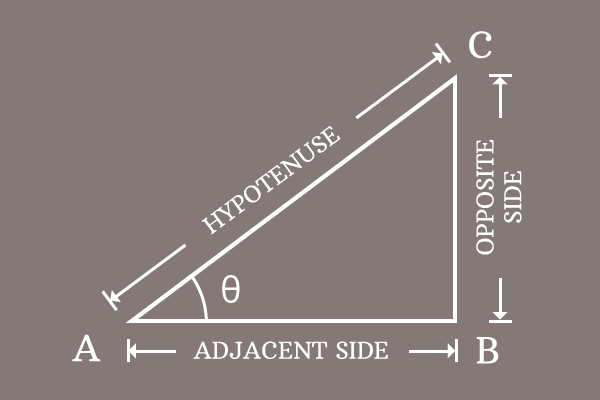# Proof of Pythagorean identity of Sine and Cosine functions

In trigonometry, the Pythagorean identity of sine and cosine functions is actually derived in mathematical form by the geometrical approach. So, let us learn how to derive this basic trigonometric identity.

### Write the relation between sides of the triangle

Construct a right triangle and it is named as $\Delta BAC$. In this triangle, the angle is denoted by theta.$\overline{AB}$, $\overline{BC}$ and $\overline{CA}$ are the adjacent side, opposite side and the hypotenuse respectively in this triangle but their corresponding lengths are written in mathematical form as $AB$, $BC$ and $CA$.

According to the Pythagorean Theorem, write the relationship between the three sides of this right triangle in mathematical form.

${AC}^2 = {AB}^2+{BC}^2$

Remember this equation and we are going to use it for proving the Pythagorean identity of sine and cosine functions.

### Express the sides in trigonometric functions

As per the right triangle $BAC$, write the lengths of the sides in terms of the sine and cosine functions. In order to do it, we have to express the sine and cosine functions firstly in their ratio form.

$\sin{\theta} \,=\, \dfrac{BC}{AC}$

$\implies$ $BC = AC \times \sin{\theta}$

$\cos{\theta} \,=\, \dfrac{AB}{AC}$

$\implies$ $AB = AC \times \cos{\theta}$

### Express the equation in trigonometric form

In the above step, we have derived the lengths of the opposite and adjacent sides in trigonometric functions.

1. $AB = AC \times \cos{\theta}$
2. $BC = AC \times \sin{\theta}$

In the first step, we have written the Pythagorean theorem in terms of the sides of the right triangle $BAC$.

${AC}^2 = {AB}^2+{BC}^2$

Now, substitute the lengths of the $\overline{AB}$ and $\overline{BC}$ in the above equation.

$\implies$ ${AC}^2 = (AC \times \cos{\theta})^2+(AC \times \sin{\theta})^2$

Each term in the right hand side of the equation can be simplified by using the power rule of a product.

$\implies$ ${AC}^2 = (AC)^2 \times (\cos{\theta})^2+(AC)^2 \times (\sin{\theta})^2$

$\implies$ ${AC}^2 = AC^2 \times \cos^2{\theta}+AC^2 \times \sin^2{\theta}$

In this equation, $AC^2$ is a common factor in the both terms of the right side expression. So, it can be taken out common from them by factorization.

$\implies$ ${AC}^2 = AC^2 \times (\cos^2{\theta}+\sin^2{\theta})$

$\implies$ $AC^2 \times (\cos^2{\theta}+\sin^2{\theta}) = {AC}^2$

$\implies$ $\cos^2{\theta}+\sin^2{\theta} = \dfrac{{AC}^2}{AC^2}$

$\implies$ $\require{cancel} \cos^2{\theta}+\sin^2{\theta} = \dfrac{\cancel{{AC}^2}}{\cancel{AC^2}}$

$\implies$ $\cos^2{\theta}+\sin^2{\theta} = 1$

Therefore, it is proved that the sum of cos squared angle and sin squared angle is equal to one and it is called the Pythagorean identity of sine and cosine functions. It is also written as follows.

$\,\,\, \therefore \,\,\,\,\,\,$ $\sin^2{\theta}+\cos^2{\theta} \,=\, 1$

A best free mathematics education website that helps students, teachers and researchers.

###### Maths Topics

Learn each topic of the mathematics easily with understandable proofs and visual animation graphics.

###### Maths Problems

A math help place with list of solved problems with answers and worksheets on every concept for your practice.

Learn solutions

###### Subscribe us

You can get the latest updates from us by following to our official page of Math Doubts in one of your favourite social media sites.